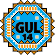General Utility Library for C++14  2.9
gul14::MinMax< DataT, typename, typename > Struct Template Reference

## Detailed Description

### template<typename DataT, typename = void, typename = std::enable_if_t<std::is_arithmetic<DataT>::value>> struct gul14::MinMax< DataT, typename, typename >

Object that is designed to holds two values: minimum and maximum of something.

DataT must be an arithmetic type.

Default constructed the members have these possibly useful values:

• min: Not-a-Number or maximum value representable with DataT
• max: Not-a-Number or minimum value representable with DataT
Template Parameters
 DataT Type of the contained values

`#include <statistics.h>`

## Public Attributes

DataT min { std::numeric_limits<DataT>::max() }
Minimum value.

DataT max { std::numeric_limits<DataT>::lowest() }
Maximum value.

The documentation for this struct was generated from the following file: